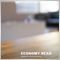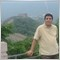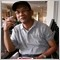# How can I solve "invalid volume" problem on my expert advisor?625

Hi, everyone,

But there is an "invalid volume" problem in my coding.

Below is the method I learn and think at mql5 articles.

```//+------------------------------------------------------------------+
//| Check the correctness of the order volume                        |
//+------------------------------------------------------------------+
bool CheckVolumeValue(double volume,string &description)
{
//--- minimal allowed volume for trade operations
double min_volume=SymbolInfoDouble(Symbol(),SYMBOL_VOLUME_MIN);
if(volume<min_volume)
{
description=StringFormat("Volume is less than the minimal allowed SYMBOL_VOLUME_MIN=%.2f",min_volume);
return(false);
}

//--- maximal allowed volume of trade operations
double max_volume=SymbolInfoDouble(Symbol(),SYMBOL_VOLUME_MAX);
if(volume>max_volume)
{
description=StringFormat("Volume is greater than the maximal allowed SYMBOL_VOLUME_MAX=%.2f",max_volume);
return(false);
}

//--- get minimal step of volume changing
double volume_step=SymbolInfoDouble(Symbol(),SYMBOL_VOLUME_STEP);

int ratio=(int)MathRound(volume/volume_step);
if(MathAbs(ratio*volume_step-volume)>0.0000001)
{
description=StringFormat("Volume is not a multiple of the minimal step SYMBOL_VOLUME_STEP=%.2f, the closest correct volume is %.2f",
volume_step,ratio*volume_step);
return(false);
}
description="Correct volume value";
return(true);
}```

I am going to put above code into "on Init" function of my expert advisor.

Lot size of order at my expert advisor is 0.1 and volume is 0.01.

Is it right?

Thanks to everyone.1564

You need to limit the lot size to me always >= Min lot that broker allow and <= Max lot.

Also, always normalize lot size to lot digits as following ...

```   double maxlot = MarketInfo(Symbol(),MODE_MAXLOT);
double minlot = MarketInfo(Symbol(),MODE_MINLOT);
if(minlot==0.001) LotDigits=3;
if(minlot==0.01) LotDigits=2;
if(minlot==0.1) LotDigits=1;
if(minlot==1) LotDigits=0;

if (Lot>=maxlot) Lot = maxlot;
if (Lot<=minlot) Lot = minlot;

if(!UseMM) Lot = Lots;
return(NormalizeDouble(Lot,LotDigits));```

The above is not a ready code to use, but as you are a coder you will understand what I mean :)57558

Chang Suk Chung:

Hi, everyone,

But there is an "invalid volume" problem in my coding.

Below is the method I learn and think at mql5 articles.

I am going to put above code into "on Init" function of my expert advisor.

Lot size of order at my expert advisor is 0.1 and volume is 0.01.

Is it right?

Thanks to everyone.

Your code just do the check volume (Lots Size), but did not make the correction volume size, if CheckVolumeValue() return false.

```
//---------- For example --------//

if(!CheckVolumeValue(vol,descript))
{
if(vol<SymbolInfoDouble(Symbol(),SYMBOL_VOLUME_MIN))
vol=NormalizeDouble(SymbolInfoDouble(Symbol(),SYMBOL_VOLUME_MIN),2);
if(vol>SymbolInfoDouble(Symbol(),SYMBOL_VOLUME_MAX))
vol=NormalizeDouble(SymbolInfoDouble(Symbol(),SYMBOL_VOLUME_MAX),2);
}
//------------------------```625

Osama Shaban:

You need to limit the lot size to me always >= Min lot that broker allow and <= Max lot.

Also, always normalize lot size to lot digits as following ...

The above is not a ready code to use, but as you are a coder you will understand what I mean :)

Thanks very much.625

Roberto Jacobs:

Your code just do the check volume (Lots Size), but did not make the correction volume size, if CheckVolumeValue() return false.

Osama Shaban:

You need to limit the lot size to me always >= Min lot that broker allow and <= Max lot.

Also, always normalize lot size to lot digits as following ...

The above is not a ready code to use, but as you are a coder you will understand what I mean :)

Then I have another guestion.

How can I confirm on whether or not there are or no "invalid volume"error?

Below is the journal at strategy tester visualization.

And This is MT5 platform journal at random delay, every tick based real ticks, \$1 deposit and leverage 100:1.

But I can't find "invalid volume".

How can I confirm "invalid volume" error?

Thanks very much.

God bless you!57558

Chang Suk Chung:

Then I have another guestion.

How can I confirm on whether or not there are or no "invalid volume"error?

Below is the journal at strategy tester visualization.

And This is MT5 platform journal at random delay, every tick based real ticks, \$1 deposit and leverage 100:1.

But I can't find "invalid volume".

How can I confirm "invalid volume" error?

Thanks very much.

God bless you!

Error on your EA is about problem ACCOUNT_FREEMARGIN and error There is not enough money to complete the request (TRADE_RETCODE_NO_MONEY = error 10019)

You should check the documentation MQL5 about ENUM_SYMBOL_CALC_MODE

https://www.mql5.com/en/docs/constants/environment_state/marketinfoconstants

https://www.mql5.com/en/docs/account/accountinfodouble

We can not help without seeing your EA code.Documentation on MQL5: Account Information / AccountInfoDouble
• www.mql5.com
Account Information / AccountInfoDouble - Reference on algorithmic/automated trading language for MetaTrader 5625

Roberto Jacobs:

Error on your EA is about problem ACCOUNT_FREEMARGIN and error There is not enough money to complete the request (TRADE_RETCODE_NO_MONEY = error 10019)

You should check the documentation MQL5 about ENUM_SYMBOL_CALC_MODE

https://www.mql5.com/en/docs/constants/environment_state/marketinfoconstants

https://www.mql5.com/en/docs/account/accountinfodouble